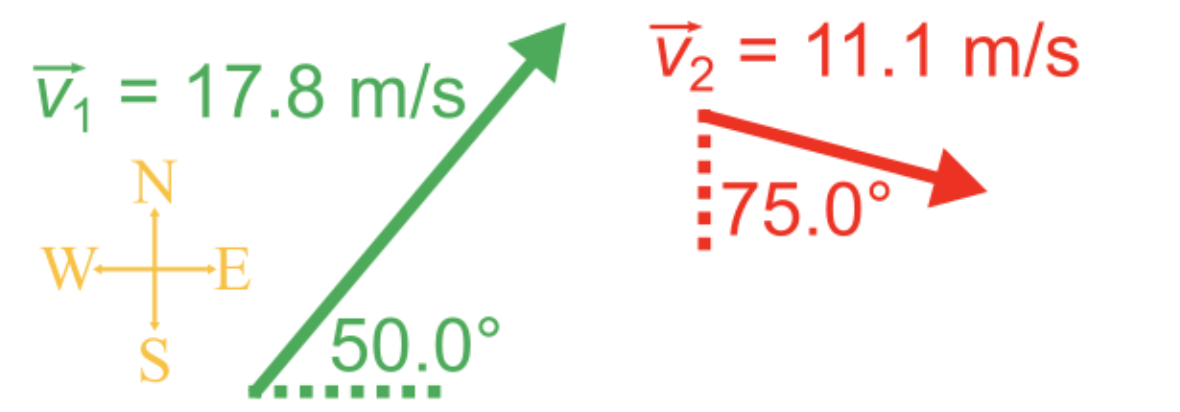# Vector components

### Vector components

#### Lessons

In this lesson, we will learn:

• What are x and y components?
• How to break a vector into its components
• Problem solving with vector components

Notes:

• Components of a vector are other vectors that add up tip-to-tail give you the original vector.
• The x and y components of a vector are the components that are pointed directly in the x and y directions, respectively, and are useful for solving problems.
• The x and y components can be found with trigonometry, since they always form a right triangle with the original vector.
Right Triangle Trigonometric Equations

$\sin(\theta) = \frac{opp.}{hyp.}$

$\cos(\theta) = \frac{adj.}{hyp.}$

$\tan(\theta) = \frac{opp.}{adj.}$

$a^{2}+b^{2}=c^{2}$ (Pythagorean theorem)

$\theta$: angle, in degrees (°)

$opp.$: side opposite angle

$adj.$: side adjacent angle

$hyp.$: hypotenuse of triangle (longest side, side opposite 90° angle)

$a$ and $b$: non-hypotenuse sides of triangle

$c$: hypotenuse of triangle

• Introduction
Introduction to vector components:
• What are x and y components?
• How to break a vector into its components

• 1.
Break a displacement into vector components

A boat sails 33.0 km at 55.0° north of west. What are the north and west components of its displacement?

• 2.
Use components of velocity for calculations

A plane that is taking off travels at 67.0 m/s at a 15.0° angle above the horizontal.

i. How long does it take the plane to reach its cruising altitude of 12.0 km?

If the plane is headed east, how far east has it travelled in this time?

• 3.
Add two 2D vectors using components

Solve the equation $\vec{v}_{1} + \vec{v}_{2} = \vec{v}_{res}$ by breaking $\vec{v}_{1}$ and $\vec{v}_{2}$ into their x and y components.